# [Harvard CS264] 09 - Machine Learning on Big Data: Lessons Learned from Google Projects (Max Lin, Google Research)

31 Mar 2011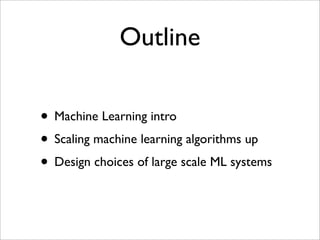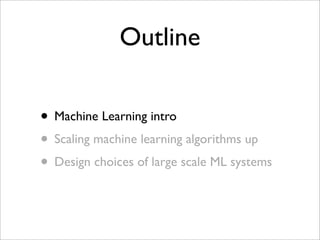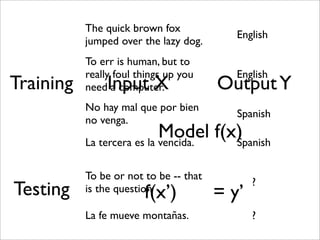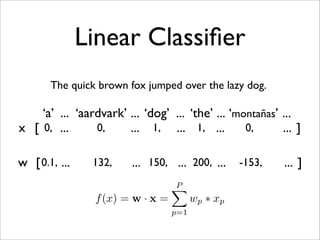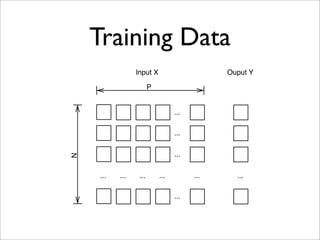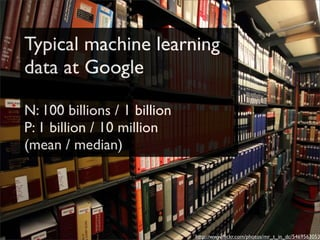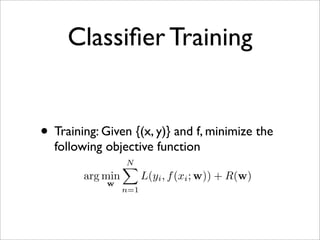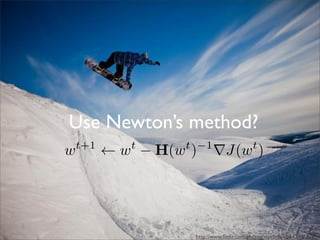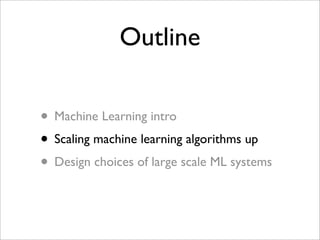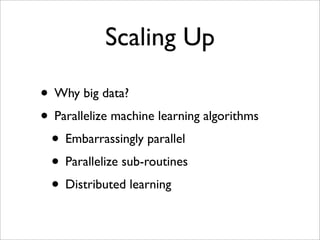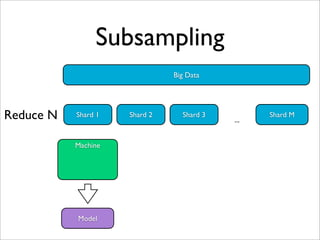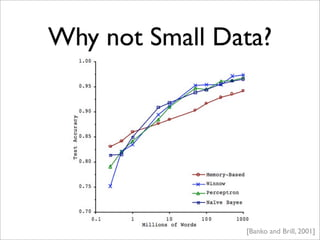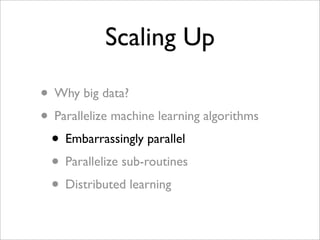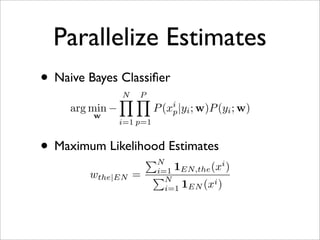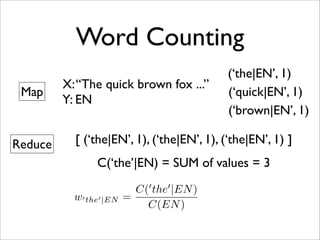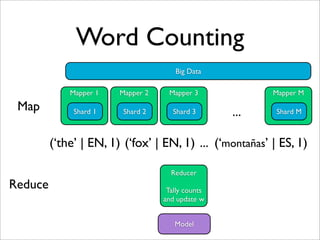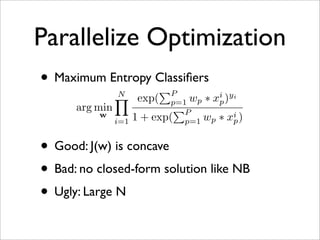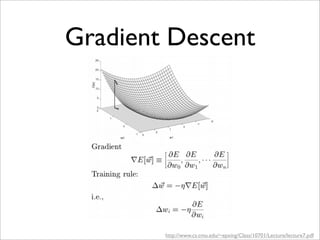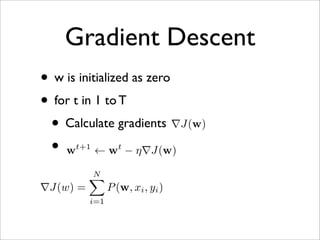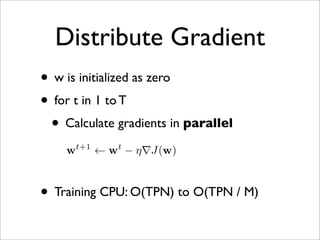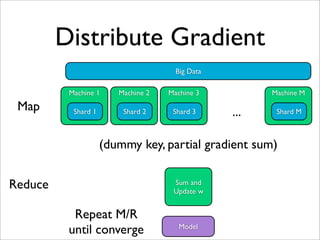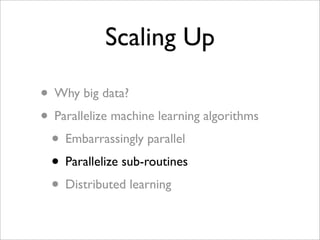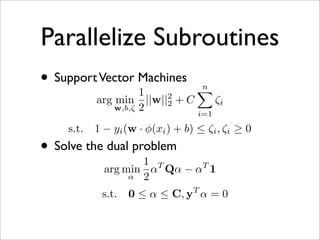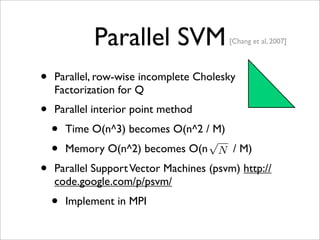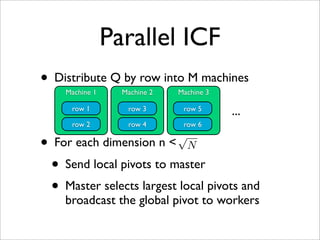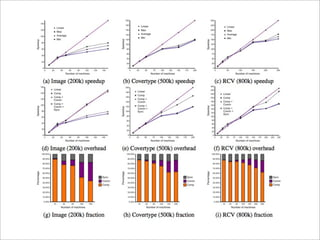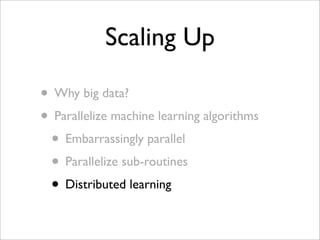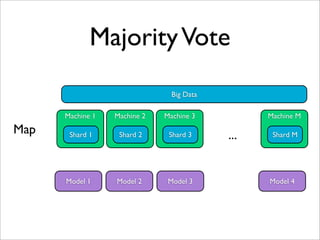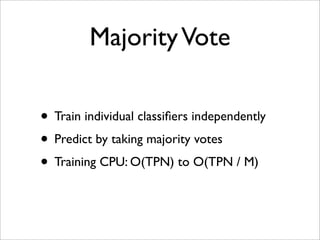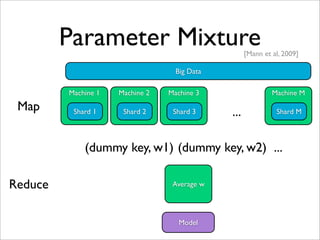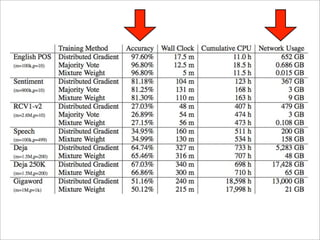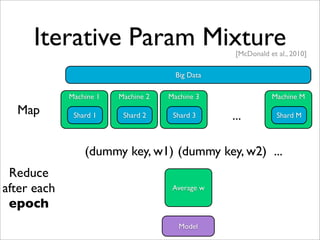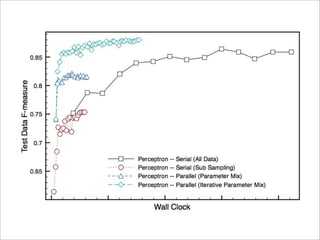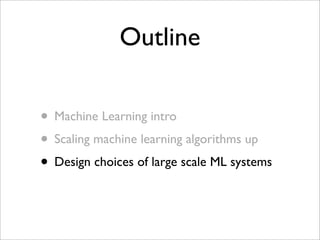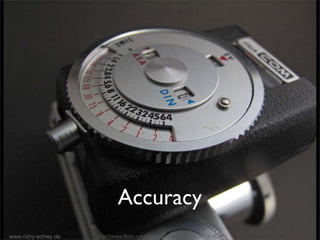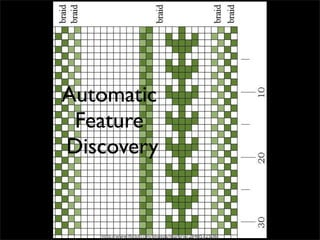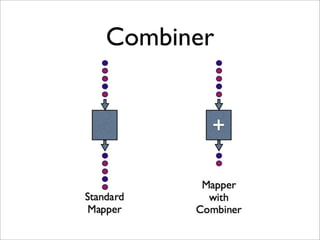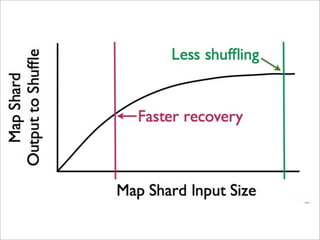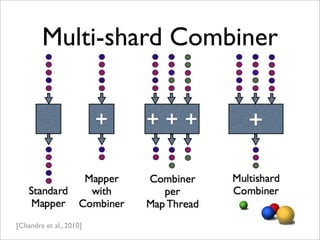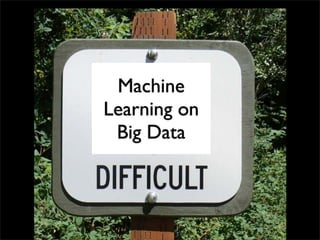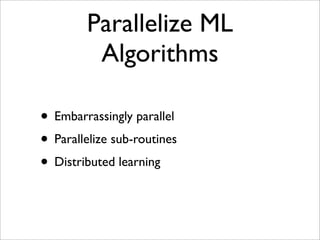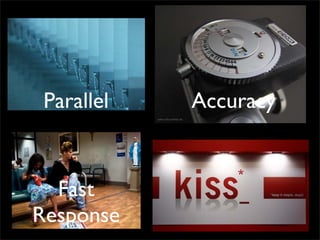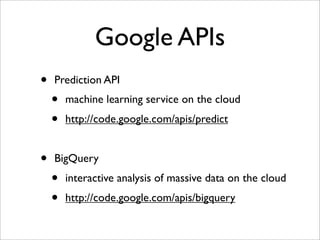1 sur 60

### [Harvard CS264] 09 - Machine Learning on Big Data: Lessons Learned from Google Projects (Max Lin, Google Research)

• 1. Machine Learning on Big Data Lessons Learned from Google Projects Max Lin Software Engineer | Google Research Massively Parallel Computing | Harvard CS 264 Guest Lecture | March 29th, 2011
• 2. Outline • Machine Learning intro • Scaling machine learning algorithms up • Design choices of large scale ML systems
• 3. Outline • Machine Learning intro • Scaling machine learning algorithms up • Design choices of large scale ML systems
• 4. “Machine Learning is a study of computer algorithms that improve automatically through experience.”
• 10. The quick brown fox English jumped over the lazy dog. To err is human, but to really foul things up you English Training Input X need a computer. Output Y No hay mal que por bien Spanish no venga. Model f(x) La tercera es la vencida. Spanish To be or not to be -- that ? Testing f(x’) is the question = y’ La fe mueve montañas. ?
• 11. Linear Classiﬁer The quick brown fox jumped over the lazy dog. ‘a’ ... ‘aardvark’ ... ‘dog’ ... ‘the’ ... ‘montañas’ ... x [ 0, ... 0, ... 1, ... 1, ... 0, ... ] w [ 0.1, ... 132, ... 150, ... 200, ... -153, ... ] P f (x) = w · x = wp ∗ xp p=1
• 12. Training Data Input X Ouput Y P ... ... ... N ... ... ... ... ... ... ...
• 13. Typical machine learning data at Google N: 100 billions / 1 billion P: 1 billion / 10 million (mean / median) http://www.ﬂickr.com/photos/mr_t_in_dc/5469563053
• 14. Classiﬁer Training • Training: Given {(x, y)} and f, minimize the following objective function N arg min L(yi , f (xi ; w)) + R(w) w n=1
• 15. Use Newton’s method? t+1 t t −1 t w ← w − H(w ) ∇J(w ) http://www.ﬂickr.com/photos/visitﬁnland/5424369765/
• 16. Outline • Machine Learning intro • Scaling machine learning algorithms up • Design choices of large scale ML systems
• 17. Scaling Up • Why big data? • Parallelize machine learning algorithms • Embarrassingly parallel • Parallelize sub-routines • Distributed learning
• 18. Subsampling Big Data Reduce N Shard 1 Shard 2 Shard 3 ... Shard M Machine Model
• 19. Why not Small Data? [Banko and Brill, 2001]
• 20. Scaling Up • Why big data? • Parallelize machine learning algorithms • Embarrassingly parallel • Parallelize sub-routines • Distributed learning
• 21. Parallelize Estimates • Naive Bayes Classiﬁer N P i arg min − P (xp |yi ; w)P (yi ; w) w i=1 p=1 • Maximum Likelihood Estimates N i i=1 1EN,the (x ) wthe|EN = N i=1 1EN (xi )
• 22. Word Counting (‘the|EN’, 1) X: “The quick brown fox ...” Map (‘quick|EN’, 1) Y: EN (‘brown|EN’, 1) Reduce [ (‘the|EN’, 1), (‘the|EN’, 1), (‘the|EN’, 1) ] C(‘the’|EN) = SUM of values = 3 C( the |EN ) w the |EN = C(EN )
• 23. Word Counting Big Data Mapper 1 Mapper 2 Mapper 3 Mapper M Map Shard 1 Shard 2 Shard 3 ... Shard M (‘the’ | EN, 1) (‘fox’ | EN, 1) ... (‘montañas’ | ES, 1) Reducer Reduce Tally counts and update w Model
• 24. Parallelize Optimization • Maximum Entropy Classiﬁers P N i yi exp( p=1 wp ∗ xp ) arg min P w i=1 1 + exp( p=1 wp ∗ xi ) p • Good: J(w) is concave • Bad: no closed-form solution like NB • Ugly: Large N
• 26. Gradient Descent • w is initialized as zero • for t in 1 to T • Calculate gradients ∇J(w) • w ← w − η∇J(w) t+1 t N ∇J(w) = P (w, xi , yi ) i=1
• 27. Distribute Gradient • w is initialized as zero • for t in 1 to T • Calculate gradients in parallel wt+1 ← wt − η∇J(w) • Training CPU: O(TPN) to O(TPN / M)
• 28. Distribute Gradient Big Data Machine 1 Machine 2 Machine 3 Machine M Map Shard 1 Shard 2 Shard 3 ... Shard M (dummy key, partial gradient sum) Reduce Sum and Update w Repeat M/R until converge Model
• 29. Scaling Up • Why big data? • Parallelize machine learning algorithms • Embarrassingly parallel • Parallelize sub-routines • Distributed learning
• 30. Parallelize Subroutines • Support Vector Machines 1 n 2 arg min ||w||2 +C ζi w,b,ζ 2 i=1 s.t. 1 − yi (w · φ(xi ) + b) ≤ ζi , ζi ≥ 0 • Solve the dual problem 1 T arg min α Qα − αT 1 α 2 s.t. 0 ≤ α ≤ C, yT α = 0
• 31. The computational cost for the Primal- Dual Interior Point Method is O(n^3) in time and O(n^2) in memory http://www.ﬂickr.com/photos/sea-turtle/198445204/
• 32. Parallel SVM [Chang et al, 2007] • Parallel, row-wise incomplete Cholesky Factorization for Q • Parallel interior point method • Time O(n^3) becomes O(n^2 / M) √ • Memory O(n^2) becomes O(n N / M) • Parallel Support Vector Machines (psvm) http:// code.google.com/p/psvm/ • Implement in MPI
• 33. Parallel ICF • Distribute Q by row into M machines Machine 1 Machine 2 Machine 3 row 1 row 3 row 5 ... row 2 row 4 row 6 • For each dimension n N √ • Send local pivots to master • Master selects largest local pivots and broadcast the global pivot to workers
• 35. Scaling Up • Why big data? • Parallelize machine learning algorithms • Embarrassingly parallel • Parallelize sub-routines • Distributed learning
• 36. Majority Vote Big Data Machine 1 Machine 2 Machine 3 Machine M Map Shard 1 Shard 2 Shard 3 ... Shard M Model 1 Model 2 Model 3 Model 4
• 37. Majority Vote • Train individual classiﬁers independently • Predict by taking majority votes • Training CPU: O(TPN) to O(TPN / M)
• 38. Parameter Mixture [Mann et al, 2009] Big Data Machine 1 Machine 2 Machine 3 Machine M Map Shard 1 Shard 2 Shard 3 ... Shard M (dummy key, w1) (dummy key, w2) ... Reduce Average w Model
• 39. Much Less network usage than distributed gradient descent O(MN) vs. O(MNT) ttp://www.ﬂickr.com/photos/annamatic3000/127945652/
• 41. Iterative Param Mixture [McDonald et al., 2010] Big Data Machine 1 Machine 2 Machine 3 Machine M Map Shard 1 Shard 2 Shard 3 ... Shard M (dummy key, w1) (dummy key, w2) ... Reduce after each Average w epoch Model
• 43. Outline • Machine Learning intro • Scaling machine learning algorithms up • Design choices of large scale ML systems
• 44. Scalable http://www.ﬂickr.com/photos/mr_t_in_dc/5469563053
• 45. Parallel http://www.ﬂickr.com/photos/aloshbennett/3209564747/
• 46. Accuracy http://www.ﬂickr.com/photos/wanderlinse/4367261825/